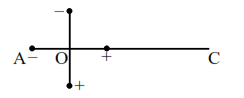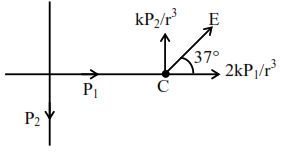# Two ideal electric dipoles`
Question:

Two ideal electric dipoles $\mathrm{A}$ and $\mathrm{B}$, having their dipole moment $p_{1}$ and $p_{2}$ respectively are placed on a plane with their centres at $O$ as shown in the figure. At point $\mathrm{C}$ on the axis of dipole $\mathrm{A}$, the resultant electric field is making an angle of $37^{\circ}$ with the axis. The ratio of the dipole moment of $\mathrm{A}$ and $\mathrm{B}, \frac{\mathrm{p}_{1}}{\mathrm{p}_{2}}$ is : $\left(\right.$ take $\left.\sin 37^{\circ}=\frac{3}{5}\right)$1. $\frac{3}{8}$

2. $\frac{3}{2}$

3. $\frac{2}{3}$

4. $\frac{4}{3}$

Correct Option: , 3

Solution:$\tan 37^{\circ}=\frac{3}{4}=\frac{\frac{\mathrm{kP}_{2}}{\mathrm{r}^{3}}}{\frac{2 \mathrm{kP}_{1}}{\mathrm{r}^{3}}}=\frac{\mathrm{P}_{2}}{2 \mathrm{P}_{1}}=\frac{3}{4}$

$\frac{\mathrm{P}_{2}}{\mathrm{P}_{1}}=\frac{3}{2}$

$\frac{\mathrm{P}_{1}}{\mathrm{P}_{2}}=\frac{2}{3}$